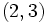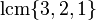# Symmetric group:S6

View a complete list of particular groups (this is a very huge list!)[SHOW MORE]

## Definition

The symmetric group$S_6$, called the symmetric group of degree six, is defined in the following equivalent ways:

## Arithmetic functions

Want to compare and contrast arithmetic function values with other groups of the same order? Check out groups of order 720#Arithmetic functions

### Basic arithmetic functions

Function Value Similar groups Explanation for function value
order (number of elements, equivalently, cardinality or size of underlying set) 720 groups with same order As$\!S_n, n = 6:$$n! = 6! = 6 \cdot 5 \cdot 4 \cdot 3 \cdot 2 \cdot 1 = 720$
As$\! Sp(2m,q)$,$\! m = 2, q = 2$ (see order formulas for symplectic groups):$q^{m^2}\prod_{i=1}^m (q^{2i} - 1)$ which becomes$2^4(2^2 - 1)(2^4 - 1) = 16 \cdot 3 \cdot 15 = 720$
exponent 60 groups with same order and exponent | groups with same exponent As$\! S_n, n = 6:$$\operatorname{lcm} \{ 1,2,3,4,5,6 \} = 60$
derived length -- not a solvable group
nilpotency class -- not a nilpotent group
Frattini length 1 groups with same order and Frattini length | groups with same Frattini length Frattini-free group; see also symmetric groups are Frattini-free
minimum size of generating set 2 groups with same order and minimum size of generating set | groups with same minimum size of generating set$\! (1,2), (1,2,3,4,5,6)$; see also symmetric group on a finite set is 2-generated
subgroup rank of a group 3 groups with same order and subgroup rank of a group | groups with same subgroup rank of a group The group elementary abelian group:E8 can be embedded in this group as$\langle (1,2), (3,4), (5,6) \rangle$
max-length of a group 6 groups with same order and max-length of a group | groups with same max-length of a group This is a rare example of a small group whose max-length is less than the sum of the exponents of all prime divisors
composition length 2 groups with same order and composition length | groups with same composition length The subgroup alternating group:A6 is simple and normal (see alternating groups are simple) and the quotient is simple (cyclic of order two)
chief length 2 groups with same order and chief length | groups with same chief length The subgroup alternating group:A6 is simple and normal (see alternating groups are simple) and the quotient is simple (cyclic of order two)

### Arithmetic functions of a counting nature

Function Value Similar groups Explanation for function value
number of conjugacy classes 11 groups with same order and number of conjugacy classes | groups with same number of conjugacy classes As$\! S_n, n = 6:$ the number of conjugacy classes is$p(n) = p(6) = 11$, where$p$ is the number of unordered integer partitions; see cycle type determines conjugacy class.
As$Sp(4,q), q = 2$ (even):$q^2 + 2q + 3 = 2^2 + 2(2) + 3 = 11$. More here
See element structure of symmetric group:S6
number of conjugacy classes of subgroups 56 groups with same order and number of conjugacy classes of subgroups | groups with same number of conjugacy classes of subgroups
number of subgroups 1455 groups with same order and number of subgroups | groups with same number of subgroups

## Group properties

Property Satisfied? Explanation
abelian group No$(1,2)$ and$(2,3)$ don't commute. In fact,$S_n$ is non-abelian for$n \ge 3$.
nilpotent group No
solvable group No
simple group No has alternating group:A6 as a proper nontrivial normal subgroup
almost simple group Yes sandwiched between the simple group alternating group:A6 and the automorphism group thereof. See also symmetric groups are almost simple.
quasisimple group No
one-headed group Yes alternating group:A6 is the unique maximal normal subgroup
monolithic group Yes alternating group:A6 is the unique minimal normal subgroup
T-group Yes
rational representation group Yes See symmetric groups are rational representation, linear representation theory of symmetric groups
rational group Yes See symmetric groups are rational
N-group Yes See classification of symmetric groups that are N-groups

## Elements

Further information: element structure of symmetric group:S6

### Up to conjugacy

For convenience, we take the underlying set here as$\{ 1,2,3,4,5,6 \}$.

There are eleven conjugacy classes, corresponding to the unordered integer partitions of$6$ (for more information, refer cycle type determines conjugacy class).

Partition Partition in grouped form Verbal description of cycle type Representative element Size of conjugacy class Formula for size Even or odd? If even, splits? If splits, real in alternating group? Element orders Formula calculating element order
1 + 1 + 1 + 1 + 1 + 1 1 (6 times) six fixed points$()$ -- the identity element 1$\! \frac{6!}{(1)^6(6!)}$ even; no 1$\operatorname{lcm}\{ 1 \}$
2 + 1 + 1 + 1 + 1 2 (1 time), 1 (4 times) transposition, four fixed points$(1,2)$ 15$\! \frac{6!}{(2)(1)^4(4!)}$ odd 2$\operatorname{lcm}\{ 2, 1 \}$
3 + 1 + 1 + 1 3 (1 time), 1 (3 times) one 3-cycle, three fixed points$(1,2,3)$ 40$\! \frac{6!}{(3)(1)^3(3!)}$ even; no 3$\operatorname{lcm}\{ 3,1 \}$
4 + 1 + 1 4 (1 time), 1 (2 times) one 4-cycle, two fixed points$(1,2,3,4)$ 90$\! \frac{6!}{(4)(1)^2(2!)}$ odd 4$\operatorname{lcm}\{4,1 \}$
2 + 2 + 1 + 1 2 (2 times), 1 (2 times) double transposition: two 2-cycles, two fixed points$(1,2)(3,4)$ 45$\frac{6!}{(2)^2(2!)(1)^2(2!)}$ even; no 2$\operatorname{lcm}\{ 2, 1 \}$
5 + 1 5 (1 time), 1 (1 time) one 5-cycle, one fixed point$(1,2,3,4,5)$ 144$\! \frac{6!}{(5)(1)}$ even; yes; yes 5$\operatorname{lcm} \{ 5, 1 \}$
3 + 2 + 1 3 (1 time), 2 (1 time), 1 (1 time) one 3-cycle, one 2-cycle, one fixed point$(1,2,3)(4,5)$ 120$\! \frac{6!}{(3)(2)(1)}$ odd 6$\operatorname{lcm}\{3,2,1 \}$
2 + 2 + 2 2 (3 times) triple transposition$(1,2)(3,4)(5,6)$ 15$\! \frac{6!}{(2)^3(3!)}$ odd 2$\operatorname{lcm} \{ 2 \}$
4 + 2 4 (1 time), 2 (1 time) one 4-cycle, one 2-cycle$(1,2,3,4)(5,6)$ 90$\! \frac{6!}{(4)(2)}$ even; no 4$\operatorname{lcm} \{ 4 , 2 \}$
3 + 3 3 (2 times) two 3-cycles$(1,2,3)(4,5,6)$ 40$\! \frac{6!}{(3)^2(2!)}$ even; no 3$\operatorname{lcm} \{ 3 \}$
6 6 (1 time) one 6-cycle$(1,2,3,4,5,6)$ 120$\! \frac{6!}{6}$ odd 6$\operatorname{lcm} \{ 6 \}$
Total (11 rows = 11 conjugacy classes) -- -- -- 720 (equals order of the whole group) -- odd: 360, 5 classes
even;no: 216, 5 classes
even;yes;yes: 144, 1 class
order 1: 1, order 2: 75, order 3: 80, order 4: 180, order 5: 144, order 6: 240 --

### Up to automorphism

The outer automorphism group has order two, and it swaps some conjugacy classes. Below are the equivalence classes up to automorphisms.

Partitions for cycle types in one automorphism class Representative elements for each Size of each conjugacy class Number of classes Total size Element orders
1 + 1 + 1 + 1 + 1 + 1$()$ 1 1 1 1
2 + 1 + 1 + 1 + 1, 2 + 2 + 2$(1,2)$,$(1,2)(3,4)(5,6)$ 15 2 30 2
3 + 1 + 1 + 1, 3 + 3$(1,2,3)$,$(1,2,3)(4,5,6)$ 40 2 80 3
4 + 1 + 1$(1,2,3,4)$ 90 1 90 4
4 + 2$(1,2,3,4)(5,6)$ 90 1 90 4
5 + 1$(1,2,3,4,5)$ 144 1 144 5
3 + 2 + 1, 6$(1,2,3)(4,5)$,$(1,2,3,4,5,6)$ 120 2 240 6
2 + 2 + 1 + 1$(1,2)(3,4)$ 45 1 45 2
Total (8 classes) -- -- 11 720 --

## Subgroups

Further information: subgroup structure of symmetric group:S6

### Quick summary

Item Value
Number of subgroups 1455
Compared with$S_n, n = 1,2,3,\dots$: 1, 2, 6, 30, 156, 1455, 11300, 151221, ...
Number of conjugacy classes of subgroups 56
Compared with$S_n, n = 1,2,3,\dots$: 1, 2, 4, 11, 19, 56, 96, 296, ...
Number of automorphism classes of subgroups 37
Compared with$S_n, n = 1,2,3,\dots$: 1, 2, 4, 11, 19, 37, 96, 296, ...
Isomorphism classes of Sylow subgroups and the corresponding Sylow numbers and fusion systems 2-Sylow: direct product of D8 and Z2 (order 16), Sylow number is 45
3-Sylow: elementary abelian group:E9 (order 9), Sylow number is 10
5-Sylow: cyclic group:Z5 (order 5), Sylow number is 36
Hall subgroups No Hall subgroups other than the Sylow subgroups, whole group, and trivial subgroup. In particular, there is no$\{ 2,3 \}$-Hall subgroup,$\{ 2,5 \}$-Hall subgroup, and$\{ 3,5 \}$-Hall subgroup.
maximal subgroups maximal subgroups have order 48, 72, 120, and 360
normal subgroups The only normal subgroups are the whole group, the trivial subgroup, and alternating group:A6 as A6 in S6.

## Linear representation theory

Further information: linear representation theory of symmetric group:S6

### Summary

Item Summary
Degrees of irreducible representations over a splitting field 1,1,5,5,5,5,9,9,10,10,16
maximum: 16, lcm: 720, number: 11, sum of squares: 720
Schur index values of irreducible representations 1,1,1,1,1,1,1,1,1,1,1
Smallest ring of realization for all irreducible representations (characteristic zero)$\mathbb{Z}$ -- ring of integers
Smallest field of realization for all irreducible representations, i.e., smallest splitting field (characteristic zero)$\mathbb{Q}$ -- hence it is a rational representation group
Criterion for a field to be a splitting field Any field of characteristic not 2,3, or 5
Smallest size splitting field field:F7

### Character table

Representation/conjugacy class representative and size$()$ (size 1)$(1,2)$ (size 15)$(1,2)(3,4)(5,6)$ (size 15)$(1,2,3)$ (size 40)$(1,2,3)(4,5,6)$ (size 40)$(1,2)(3,4)$ (size 45)$(1,2,3,4)$ (size 90)$(1,2,3,4)(5,6)$ (size 90)$(1,2,3)(4,5)$ (size 120)$(1,2,3,4,5,6)$ (size 120)$(1,2,3,4,5)$ -- size 144
trivial 1 1 1 1 1 1 1 1 1 1 1
sign 1 -1 -1 1 1 1 -1 1 -1 -1 1
standard 5 3 -1 2 -1 1 1 -1 0 -1 0
product of standard and sign 5 -3 1 2 -1 1 -1 -1 0 1 0
other five-dimensional irreducible 5 -1 3 -1 2 1 1 -1 -1 0 0
other five-dimensional irreducible 5 1 -3 -1 2 1 -1 -1 1 0 0
nine-dimensional irreductible 9 3 3 0 0 1 -1 1 0 0 -1
product of nine-dimensional irreductible and sign 9 -3 -3 0 0 1 1 1 0 0 -1
second exterior power of standard 10 2 -2 1 1 -2 0 0 -1 1 0
third exterior power of standard 10 -2 2 1 1 -2 0 0 1 -1 0
sixteen-dimensional irreductible 16 0 0 -2 -2 0 0 0 0 0 1

## GAP implementation

### Group ID

This finite group has order 720 and has ID 763 among the groups of order 720 in GAP's SmallGroup library. For context, there are 840 groups of order 720. It can thus be defined using GAP's SmallGroup function as:

SmallGroup(720,763)

For instance, we can use the following assignment in GAP to create the group and name it$G$:

gap> G := SmallGroup(720,763);

Conversely, to check whether a given group$G$ is in fact the group we want, we can use GAP's IdGroup function:

IdGroup(G) = [720,763]

or just do:

IdGroup(G)

to have GAP output the group ID, that we can then compare to what we want.

### Other descriptions

Description Functions used
SymmetricGroup(6) SymmetricGroup
Sp(4,2) Sp
PSp(4,2) PSp